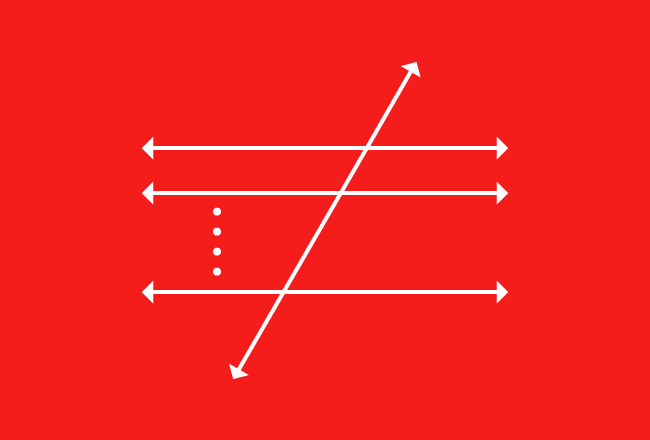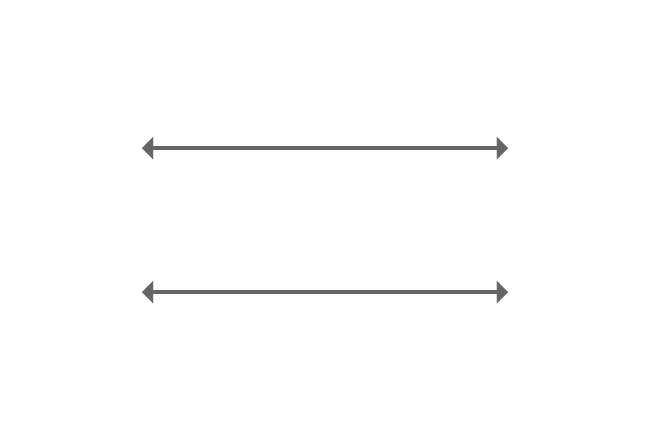# Transversal of Parallel linesA straight line that intersects either two or more parallel lines is called a transversal line of parallel lines.

A straight line often cuts two or more parallel lines geometrically in some cases. The straight line which intersects both of them at different intersecting points on a plane is called a transversal line or simply called as a transversal.

The picture represents how parallel lines cut by a transversal geometrically.

## Example

Two parallel lines cut by their transversal is a most useful geometrical concept.$\overleftrightarrow{AB}$ and $\overleftrightarrow{CD}$ are two parallel lines.

$\overleftrightarrow{XY}$ is another straight line but it intersects both parallel lines $\overleftrightarrow{AB}$ and $\overleftrightarrow{CD}$ at their intersecting points $P$ and $Q$ respectively.

The straight line $\overleftrightarrow{XY}$ is called a transversal line or a transversal of parallel lines $\overleftrightarrow{AB}$ and $\overleftrightarrow{CD}$. It is also called as parallel lines $\overleftrightarrow{AB}$ and $\overleftrightarrow{CD}$ cut by their transversal $\overleftrightarrow{XY}$.

Latest Math Topics
Jun 26, 2023
Jun 23, 2023

Latest Math Problems
Jul 01, 2023
Jun 25, 2023
###### Math Questions

The math problems with solutions to learn how to solve a problem.

Learn solutions

Practice now

###### Math Videos

The math videos tutorials with visual graphics to learn every concept.

Watch now

###### Subscribe us

Get the latest math updates from the Math Doubts by subscribing us.